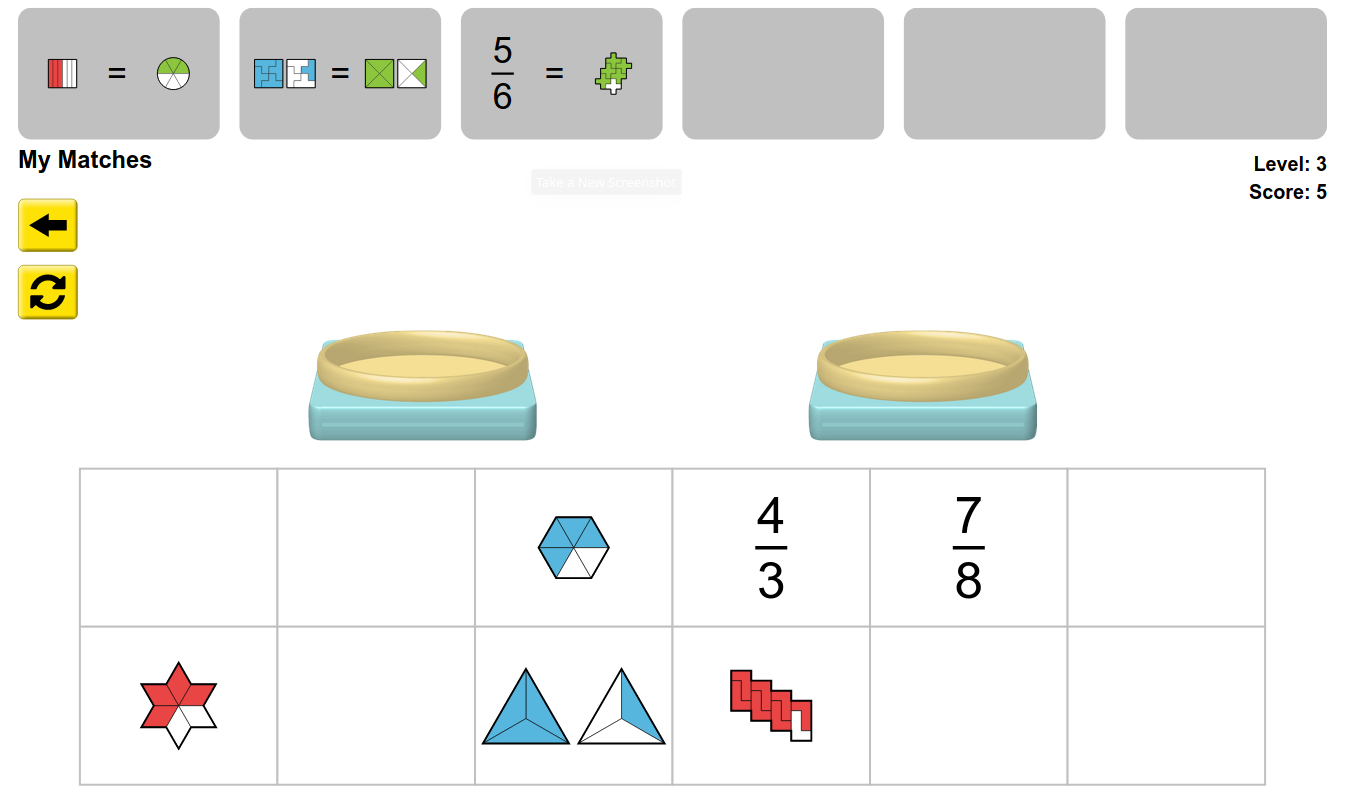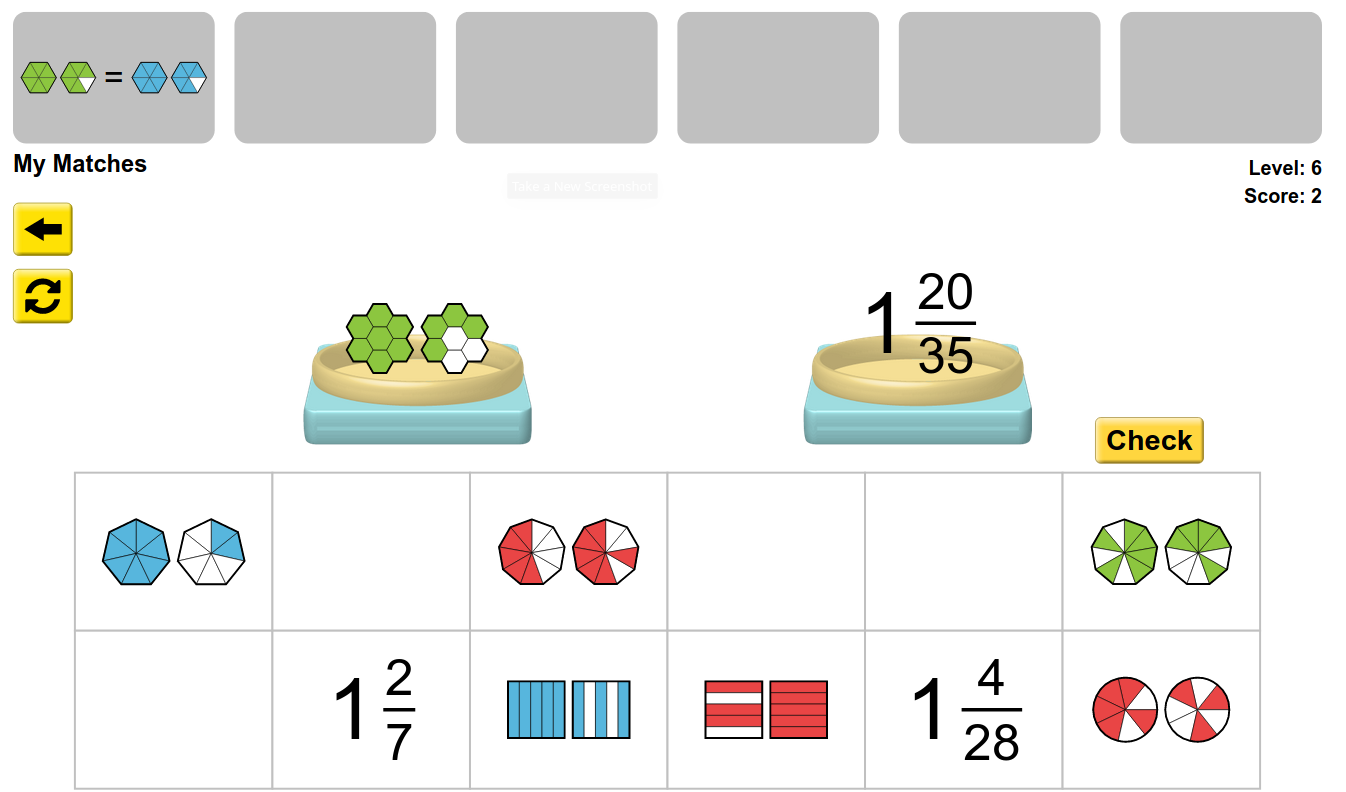MM Practice
×
Multiplication
Division
Place Value
Fractions & Decimals
Measurement
Statistics & Probability
Pre-Algebra
Money
Kindergarten
Geometry

# Fraction MatcherOnline activity and game for 1st-3th grade math

In this activity, you match visual models of fractions (or mixed numbers) with other visual models, or with the fractions written as numbers. Place the two fractions or visual models that are equal in the two pans. Then click "CHECK", and if it is correct, click "OK".

At first, you choose whether you'd like to work with FRACTIONS or MIXED NUMBERS. Either way, there are lots of difficulty levels, giving you lots of practice. In the harder levels you'll need to simplify the fractions and think of equivalent fractions. You will earn stars from completing a level.

These activities will help students to identify fractions and equivalent fractions.

Credit: This activity is created by PhET

Screenshots from the game and activity:Allow my comment to be posted on this site